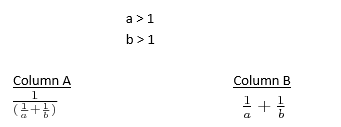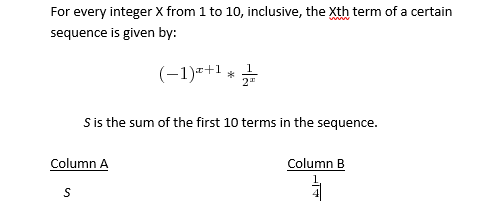+91-22-62437100 / 01 | help@reachivy.com
// //

# GRE Quantitative Comparisons Quiz 2

Quantivative comparisons is a unique question type introduced only in the GRE. In QC questions, you will be given two columns, labeled Quantity A and Quantity B. Additional information may also be provided above the two columns. You have to use the information when evaluating the problem. Quantitative Comparison question tests your ability to analyze information and figure out how to quantities compare to each other.

Instructions: Questions of this type ask you to compare two quantities — Quantity A and Quantity B — and then determine which of four statements describes the comparison.
Directions: Compare Quantity A and Quantity B, using additional information centered above the two quantities if such information is given, and select one of the following four answer choices.
A. Quantity A is greater.
B. Quantity B is greater.
C. The two quantities are equal.
D. The relationship cannot be determined from the information given.

To begin click Next

Question 1:

Working at constant rates, machine D completely makes b rolls of steel in 25 minutes and machine E completely makes b rolls of steel in one hour (b > 0).

Column A                              Column B
The number of                         The number of
rolls of steel                             rolls of steel made
made by machine                    by machine E
D in 2 hours and                      in 4 hours.
5 mins.
Question 2:

Column A                            Column B
The cost of b plums            The cost of c
at a cost of                         persimmons at a
c + 3 cents a piece              cost of b + 3 cents a piece
Question 3:
|y|-1 < -y

Column 1                             Column 2

y                                                 |y|

Question 4:

E and F are the midpoints of the opposite sides of square ABCD. The distance between one of the midpoints and any of its opposite vertices is square root of ‘10’

Column X                                Column Y
The area of region ABCD               7
Question 5:

Set A: {1, 2, 4, 8, 16, 32}
Set B: {all prime numbers less than 10}

Column A                                          Column B
The number of distinct integers           The number of integers
included in A U B divided by 6.             in A B
Question 6:

Column A                                                       Column B
The standard deviation of the sample               The standard deviation of the sample
measurements 0, 1, 2, 4, and 8.                         measurements 1, 2, 3, 5, and 9.
Question 7:
Chase's salary, which is greater than \$20,000, is 80 percent of Katy's salary. Timan's salary is 80% of Chase's salary.

Column A                                      Column B
Katy's salary                                 Timan's salary
Question 8:Question 9:You have answered all the questions in the quiz. Press Submit to view your score